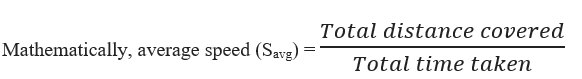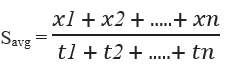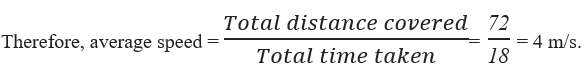# Average Speed Formula

## Average Speed Formulas - Concepts, Examples and Practice Questions

If an object covers different distances with different velocities, then average velocity of the object is a single velocity value for which if the object moves uniformly, it will cover the same distance in same interval of time.If an object covers x1,x2,x3,.......,xn distances in t1,t2,t3,.....,tn seconds respectively, thenSI unit of average speed is m/s, and is a scalar quantity, which means it has only magnitude.
Example:
An person goes from point A to point B in 10s and returns back in 8s. If distance between A and B is 36m, find the average speed of the person.

Ans:

Here total distance covered = 72m
Total time taken = 18sQuestion:
A boy covered a complete cycle along a circular path of radius 35m in 22s. Find the average speed of the boy.